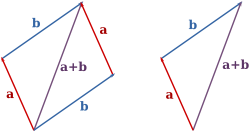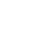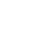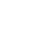# Vector Math

Physics is the science that studies motion and matter and how both work with forces and energy. It is a really big subject that has a lot of different “branches” of scientific study that include motion, sound, waves, electricity, light, sound, and even astronomy.

This science covers the tiniest particles and atoms all the way to the largest objects in the universe.

### The Basics:

A vector is defined as a property that has both direction and magnitude. Magnitude can be thought of as a length. If you draw an arrow on a piece of paper, the magnitude’s “tail” would be the starting point and the arrow top is the “head.”

When you are writing vectors on a piece of paper or in a formula they are always a letter and in boldface type. Magnitudes are always written as a the letter inside parallel lines.

An example of just vector a or b would be to write them as vector a or vector b. The magnitude of each vector would be written as ||a|| or ||b||.

Vectors can be added together so that you can figure out the resultant of both vectors. This would be written as a + b = c. When you add vectors you also include the magnitudes and the directions. This is simple addition.

Let’s say you have a short arrow that is a vector of “3” and a longer arrow that is a vector of “7”. Both arrows are going in the same direction. The resultant would be 3+7= 10.

Let’s say you have a longer arrow that is a vector of “10” going in one direction and a shorter arrow that is a vector of “3” going in the other direction. The vector going in the other direction would be a negative 3 or -3. This would be represented as 10-3 = 7.

But there are situations where all of the vectors may be going in various directions. This happens in an uneven triangle, where you have three vectors and you can use the “head-to-tail method” of addition.

You need a starting point, so you choose the tail of one vector, let’s say it’s “4” and go to its head where it meets the tail of the next vector, let’s say it’s “6” and then follow it tail to head of the third vector, let’s say it’s “5.” Using the head-to-tail method you can add 4+6+5= 15.

### Other Laws for adding Vectors

There are many shapes and sizes that could involve vectors so scientists have developed three laws to try to help in adding vectors together.

The commutative law for adding vectors basically says that it doesn’t matter which order the vectors are added together. This could be shown as a+b = b+c.

The associative law in adding vectors is very specific and relates to the addition of three or more vectors that need to be added together. In this law, it states that it doesn’t matter which vectors are added together first. This could be shown as (a+b) + d= a + (b+d)### Subtracting Vectors:

There are conditions where you will need to subtract vectors and it’s handled in the same way as adding them together, but one will be a negative number. This could be shown as a + (-b).

## Interesting Facts about vector math:

• The idea of vectors showed up in the late 19thcentury when two scientists independently developed vector analysis to express the new laws of electromagnetism. One scientist was in the U.S. and one scientist was in Britain.
• Knowing what vectors are and the math that is involved is important in the science of physics in understanding force, displacement, velocity, and acceleration.
• In real life, vector is a term that used in engineering, mathematics, and physics.
• Vectors are used in engineering to figure out where the force is stronger in a structure so that it doesn’t collapse.

### Q&A:

Do all vectors have the same direction?
no

How do you show a vector when you are writing it?
in boldface

How do you show magnitude when writing it?
the vector inside parallel lines

What is one of the methods of adding vectors in a triangle with unequal sides?
•History
•Science
•Geography
•Biography
•US History